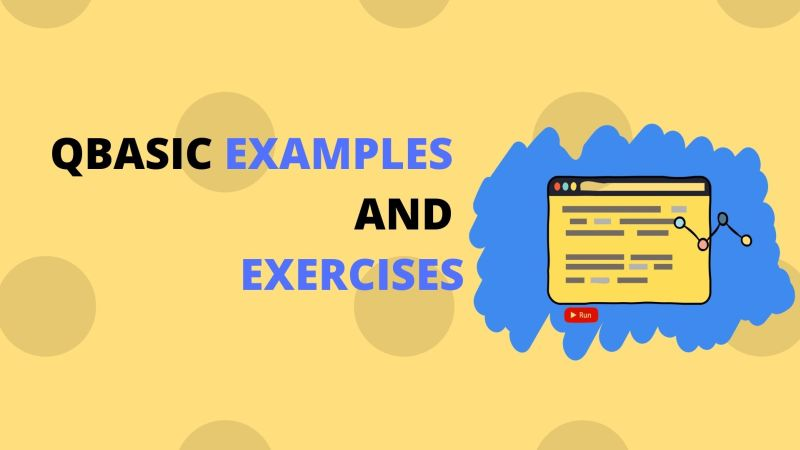# Qbasic Programming Examples and Exercises## Table Of Content

QBasic programming examples and exercises for class 6 and class 7 standard.

Qbasic sample example programs for beginners. In this article, we will see some QBasic programming examples and some simple exercises to understand how it works.

These Qbasic program examples are a beginner-level, which mostly will help you to understand the concept of a variable and some basic qbasic statements like PRINT, INPUT, CLS etc.

Here are some Qbasic commands along with the descriptions that will be used in this article.

Before getting started it is important to know the basic Qbasic statements or QBasic commands first. If you have not read about it, check: – QBasic Commands and Statements-2020

Here, we will code some simple examples which are easy to understand for class 6/ class 7 students, who have started with QBasic programming.

Here, the program codes are explained with there formulas to help you understand better.

## Exercises

EXAMPLE 1:

### Write a QBasic program to enter your name and print it.

`CLS`

`INPUT ‘Enter your name’;n\$`

`PRINT ‘The name is’;n\$`

`END`

Here since the input data is a string then the variable name ( n ) in which it is to be stored is written after INPUT command followed by \$ sign( n\$ ).

EXAMPLE 2:

### Write a qbasic program to enter your name, city, country, age and print them.

`CLS`

`INPUT ” Enter the name “;N\$`

`INPUT ” Enter the city”;C\$`

`INPUT ” Enter the country”;CO\$`

`INPUT ” Enter the age”;A`

`PRINT ” The name is “;N\$`

`PRINT ” The city is “;C\$`

`PRINT ” The country is “;CO\$`

`PRINT ” The age is “;A`

`EN`D

Here name, city, country are string so its variable name is written with sign \$ before it and since age is a number so the variable name is written directly.

Example 3:

### Write a program to find the area of a rectangle.

FORMULA: Area of a Rectangle is length x breadth. Here length is variable l and breadth is variable b. And is the variable to store the value of the result and print it as output.

`CLS`

`INPUT ” Enter the length ” ;l`

`INPUT ” Enter the breadth ” ;b`

`LET A = l*b`

`PRINT” The area of rectangle=” ;a`

`END`

Example 4:

### Write a program to find the area of the triangle.

FORMULA:  Area of triangle is ½ x base x height.

Here in this program we that variable b as base and h as height. T is the variable to store the result and print it.

`CLS`

`INPUT ” Enter the base” ;b`

`INPUT ” Enter the height” ;h`

`LET T = 1/2*b*h`

`PRINT” The area of triangle=” ;T`

`END`

Example 5:

### Write a program to find the area of the circle.

FORMULA : Area of a circle is 22/7 x radius^2. Here we use variable R as Radius. And C is the variable where we store the result.

`CLS`

`INPUT” Enter the radius ” ;R`

`LET C=22/7*R^2`

`PRINT ” The area of circle =” ;C`

`END`

Example 6:

### Write a program to find the area of the square.

FORMULA: Area of a square is = Side2  square units. Here the result is stored in variable “square”.

`CLS`

`INPUT” Enter the number” ;n`

`LET square= n^2`

`PRINT” The area of square=” ;Square`

`END`

Example 7:

### Write a program to find the volume of the box.

FORMULA: Area of a box = length x breadth x height.

The variable are lb , and h for length, breadth and height and the result will be stored in the variable volume.

`CLS`

`INPUT ” Enter the length ” ;l`

`INPUT ” Enter the breadth ” ;b`

`INPUT ” Enter the height ” ;h`

`LET volume= l*b*h`

`PRINT” The volume of box =” ;volume`

`END`

Example 8:

### Write a program to find the circumference of the circle.

FORMULA : circumference = 22/7 x Radius x 2

`CLS`

`INPUT” Enter the radius ” ;R`

`LET Circumference=22/7*R*2`

`PRINT ” The area of circle =” ;Circumference`

`END`

Example 9:

### Write a program to find out the Simple Interest.

FORMULA : Simple Interest = Principle x Rate x Time / 100

`CLS`

`INPUT ” Enter the Principal”;P`

`INPUT ” Enter the Rate”;R`

`INPUT ” Enter the Time”;T`

`LET I = P*T*R/100`

`PRINT ” The simple Interest = “;I`

`END`

Example 10:

### Write a program to find out the simple Interest and the Amount.

FORMULA : Simple Interest = Principle x Rate x Time / 100

Amount = Principle + Simple Interest

`CLS`

`INPUT ” Enter the Principal”;P`

`INPUT ” Enter the Rate”;R`

`INPUT ” Enter the Time”;T`

`LET I = P*T*R/100`

`LET A= P + I`

`PRINT ” The simple Interest = “;I`

`PRINT ” The amount=”;A`

`END`

So, these are some common Qbasic programming examples that are useful for students and new programmers.  These posts will be updated frequently with new Qbasic examples and exercises, so keep visiting for more fun programming exercises.